GCSE Maths - Casio Calculators

## GCSE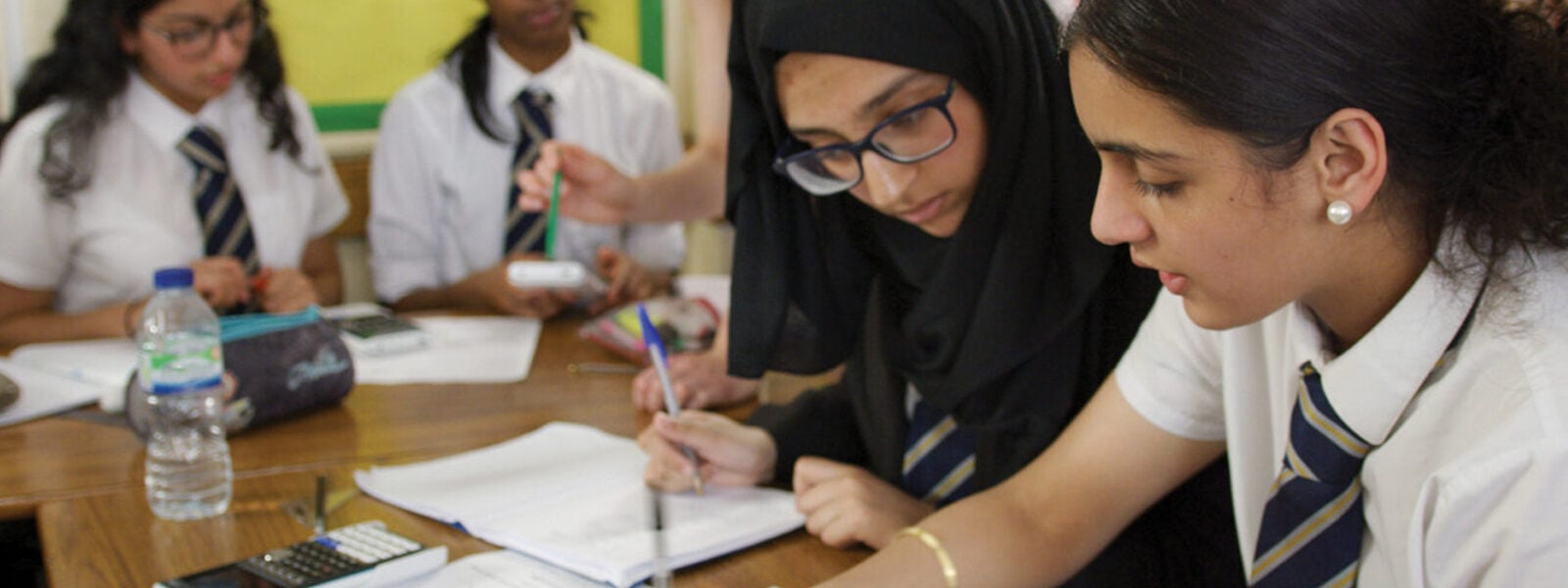## Teachers

Students will need access to at least trigonometric functions. The minimum-requirement scientific calculator is the fx-83G CW or fx-85GT CW. These have all the functionality required for GCSE. However the advanced scientific calculator fx-991CW will give students an advantage for both learning and the exam in solving equations. Please see our model comparison to evaluate these calculators.

Maths is a visual subject. A graphic calculator allows students to draw graphs and statistical charts, as well as make the same calculations as a scientific calculator. Many schools now use our fx-CG50 graphic calculator which gives a significant learning advantage, especially at the higher tier. Please see our model comparison on why it might be worth considering this calculator.

## Which Scientific Calculator?

Students will need access to at least trigonometric functions. The minimum-requirement scientific calculator is the fx-83GT CW / fx-85GT CW. This has all the functionality required for GCSE.

However, the advanced scientific calculator fx-991EX will give students an advantage for both learning and the exam in solving equations. Please see below to compare these calculators.

While the fx-83GT CW / fx-85GT CW is adequate for GCSE, the advanced scientific fx-991CW confers specific advantages. In particular, it builds students confidence in solving equations, and offers the opportunity to check exam answers. In addition to all the functionality of the basic model, it will allow students to check answers to;
• linear equations
• simultaneous linear equations
• roots and vertices of quadratics

Linear equations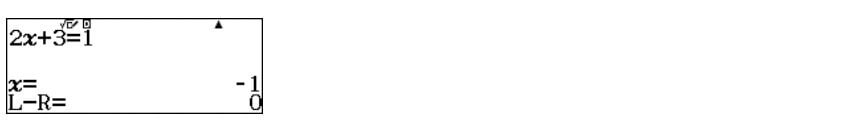Linear equations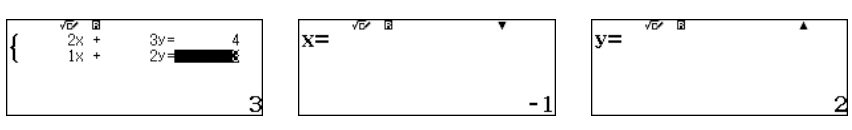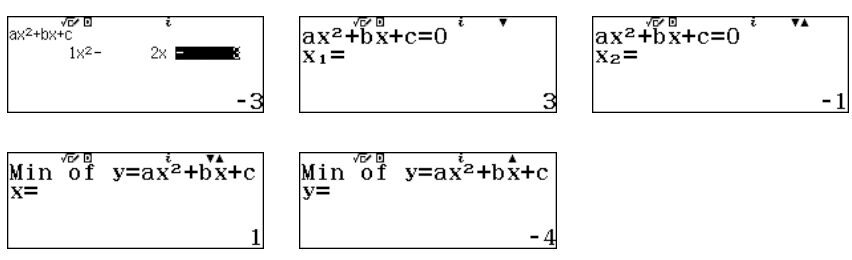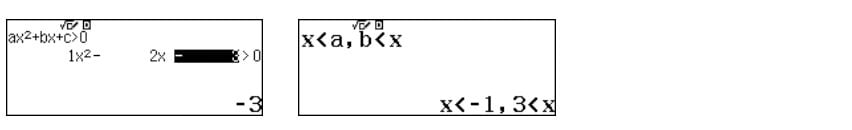## Should I use a graphic calculator?

Maths is a visual subject. A graphical calculator allows students to explore graphs. For example you could plot a graph, find the coordinates where two graphs cross or draw a data chart.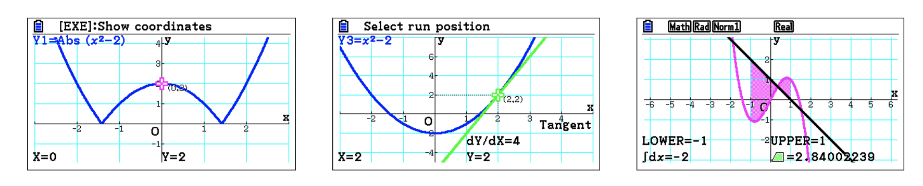Graphical calculators make learning a lot easier and more fun. They also give an advantage in exams. We recommend the fx-CG50 calculator for more confident mathematicians, perhaps those taking the Higher tier. Our graphical calculators are allowed in GCSE exams.

## Resources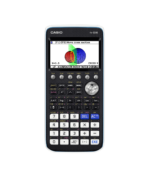Reference Leaflet
fx-CG50
Getting Started with the fx-CG50

From the main menu you can select various options which will take you to screens…How to Leaflet
fx-CG50 A-Level GCSE IB
Graph Quick Start fx-CG50

How to plot and change the view of a function on the fx-CG50. Includes tracing a…Reference Leaflet
fx-CG50

Overview of the menu functions of the fx-CG50, and the main control keys….Reference Leaflet
fx-CG50
Important Keys on the fx-CG50

Discover the important keys and how to use them. Know the effect of different re…Reference Leaflet
fx-CG50
Setting Up the fx-CG50

Change hardware settings such as display and power. Perform a memory reset. Upda…Reference Leaflet
fx-CG50
fx-CG50 Quick Start Guide

Overview of the menu functions of the fx-CG50 with examples for the key apps. Co…